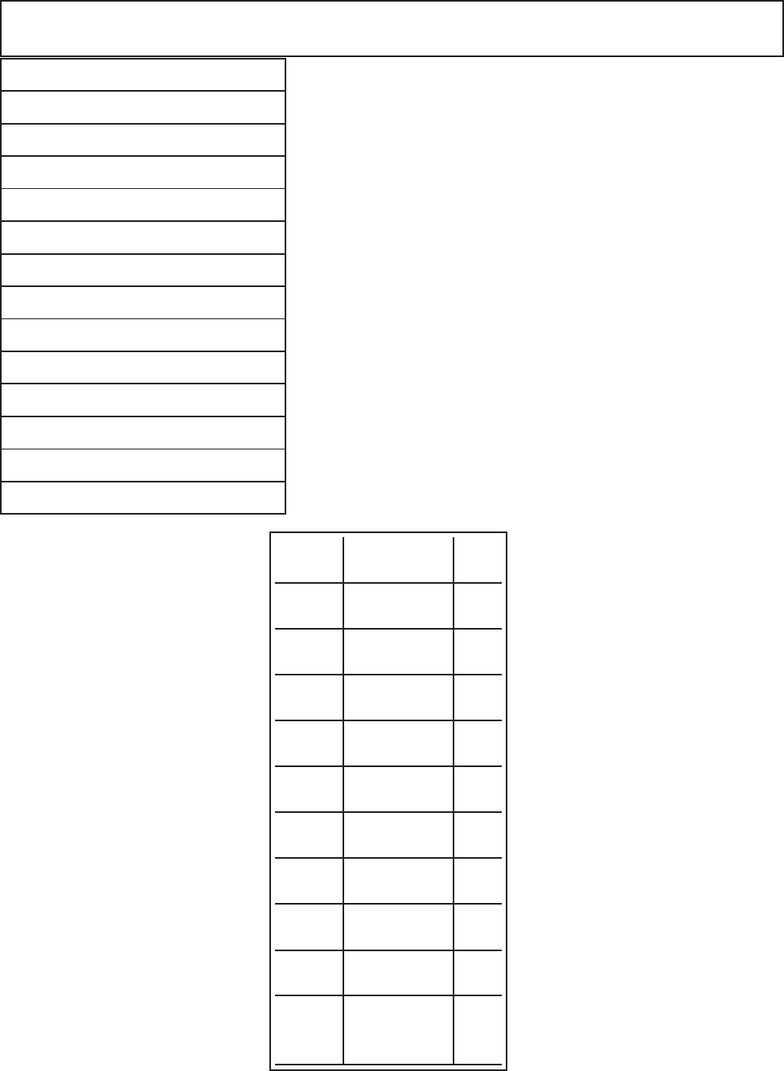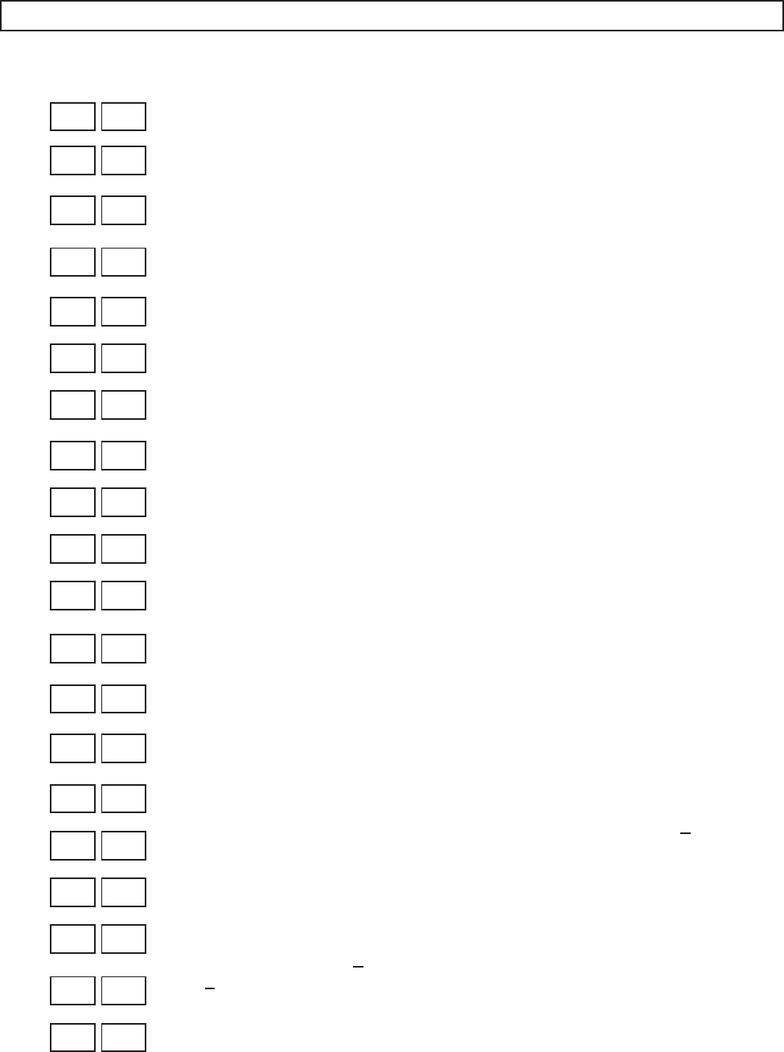Study Guides (400,000)
US (230,000)
Harvard (700)
MATH (400)
MATH 21A (100)
All (100)
Midterm

# MATH 21A Harvard 21a Fall 13Practice4Exam

Department
Mathematics
Course Code
MATH 21A
Professor
All
Study Guide
Midterm

This preview shows pages 1-2. to view the full 6 pages of the document.10/2/2013 FIRST HOURLY PRACTICE IV Math 21a, Fall 2013
Name:
MWF 9 Oliver Knill
MWF 9 Chao Li
MWF 10 Gijs Heuts
MWF 10 Yihang Zhu
MWF 11 Peter Garﬁeld
MWF 11 Matthew Woolf
MWF 12 Charmaine Sia
MWF 12 Steve Wang
MWF 14 Mike Hopkins
TTH 10 Oliver Knill
TTH 10 Francesco Cavazzani
TTH 11:30 Kate Penner
TTH 11:30 Francesco Cavazzani
Start by printing your name in the above box
and check your section in the box to the
left.
Do not detach pages from this exam packet
or unstaple the packet.
ible for the grader cannot be given credit.
Show your work. Except for problems 1-3,
we need to see details of your computation.
All functions can be diﬀerentiated arbitrarily
often unless otherwise speciﬁed.
No notes, books, calculators, computers, or
other electronic aids can be allowed.
You have 90 minutes time to complete your
work.
1 20
2 10
3 10
4 10
5 10
6 10
7 10
8 10
9 10
10 10
Total: 110
1

Only pages 1-2 are available for preview. Some parts have been intentionally blurred.Problem 1) True/False (TF) questions (20 points)
Mark for each of the 20 questions the correct letter. No justiﬁcations are needed.
1) T F The surface x2+y2+z2+ 2z= 0 is a sphere.
2) T F The length of the vector h1,2,2iis an integer.
3) T F The vector h3,4iappears as a velocity vector of the curve ~r(t) =
hcos(5t),sin(5t)i. Namely, there is a tsuch that ~r (t) = h3,4i.
4) T F If ~
Tis the unit tangent vector, ~
Nis the unit normal vector, and ~
Bis the
binormal vector, then ~
B×~
N=~
T.
5) T F The curvature of a larger circle r= 2 is greater than the curvature of a
smaller circle r= 1/2.
6) T F The surface x2y2z21 = 0 is a one sheeted hyperboloid.
7) T F The function f(x, y) = y2x2has a graph that is an elliptic paraboloid.
8) T F Let ~r(t) be a parametrization of a curve. If ~r(t) is always parallel to the
tangent vector ~r (t), then the curve is part of a line through the origin.
9) T F If Proj~
k(~u) is perpendicular to ~u, then ~u is the zero vector.
10) T F If Proj~
k(~u) is perpendicular to ~u, then Proj~
k(~u) is the zero vector.
11) T F If ~u ×~v =~
0 then ~u =~
0 or ~v =~
0.
12) T F There are two vectors ~a and ~
bsuch that the scalar projection of ~a onto ~
bis
100 times the magnitude of ~
b.
13) T F The curve ~r(t) = hcos(t), et+ 10, t2i,2t6 and the curve ~r(t) =
hcos(2t), e2t,4t2i,1t3 have the same length.
14) T F The equation ρsin(φ)2 sin(θ) = 0 in spherical coordinates deﬁnes a two
sheeted hyperboloid.
15) T F If triple scalar product of three vectors ~u, ~v, ~w is larger than |~u ×~v|then
|~w|>1.
16) T F The distance between the x-axis and the line x=y= 1 is 2.
17) T F The vector h−1,2,3iis perpendicular to the plane x2y3z= 9.
18) T F The curve ~r(t) = t3h1,2,3iis a line.
19) T F The point (1,1,3) is in spherical coordinates given by (ρ, θ, φ) =
(5, π/4,2π/3).
20) T F If the cross product satisﬁes (~v ×~w)×~v =~
0 then ~v and ~w are orthogonal.
2